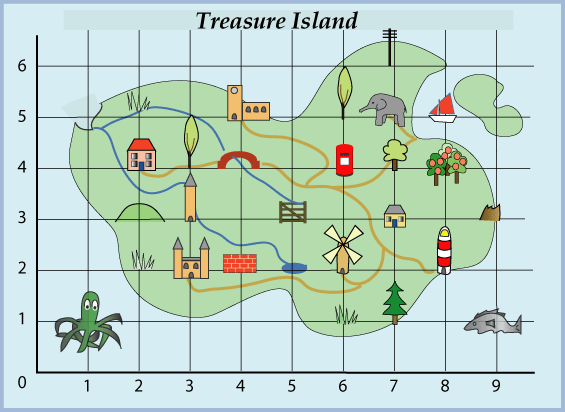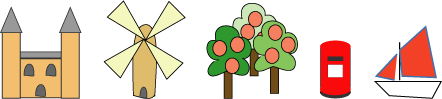## Monday, 15 October 2018

### Treasure Island Co-ordinatesWhat are the co-ordinates of these objects?Write down what you can see at the co-ordinates given below:
1. (7,5) = Elephant
2. (9,5) = Island
3. (5,3) = Gate
4. (2,3) = Hill / Mountain
5. (7,1) = Tree
6. (3,3) = Tower
7. (4,2) = Brick Wall
8. (1,1) = Octopus
The words can be used in a special way to find the coordinates of the buried treasure.
Can you work out where it is? (X,Y). (EIGHT,TWO) or (x8,y2). Lighthouse

Extension: Could you give directions to the octopus so it can get to the elephant?
Go Up to (1,5) Across to (7,5) = Elephant (EIGHT,TWO) (x8,y2)

#### 1 comment:

1.Hello Hinerangi, i like your hard work you put in to this.
keep it up.